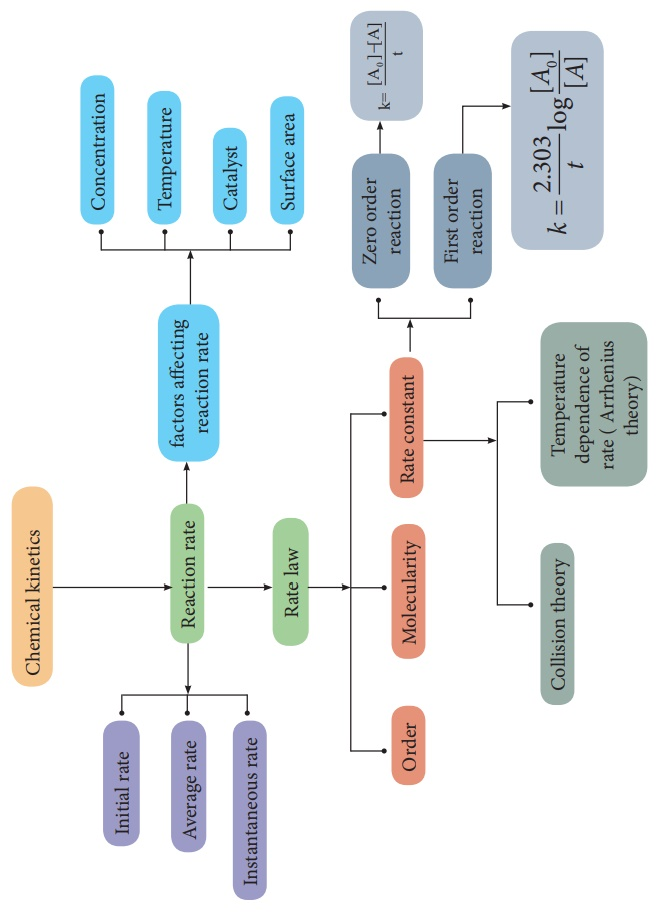Home | | Chemistry 12th Std | Chemical Kinetics: Summary

# Chemical Kinetics: Summary

Chemical kinetics is the study of the rate and the mechanism of chemical reactions, proceeding under given conditions of temperature, pressure, concentration etc.

Summary

·           Chemical kinetics is the study of the rate and the mechanism of chemical reactions, proceeding under given conditions of temperature, pressure, concentration etc.

·           The change in the concentration of the species involved in a chemical reaction per unit time gives the rate of a reaction.

·           The rate of the reaction, at a particular instant during the reaction is called the instantaneous rate. The shorter the time period, we choose, the closer we approach to the instantaneous rate,

·           The rate represents the speed at which the reactants are converted into products at any instant.

·           The rate constant is a proportionality constant and It is equal to the rate of reaction, when the concentration of each of the reactants in unity

·           Molecularity of a reaction is the total number of reactant species that are involved in an elementary step.

·           The half life of a reaction is defined as the time required for the reactant concentration to reach one half its initial value. For a first order reaction, the half life is a constant i.e., it does not depend on the initial concentration.

·           According to collision theory, chemical reactions occur as a result of collisions between the reacting molecules.

·           Generally, the rate of a reaction increase with increasing temperature. However, there are very few exceptions. The magnitude of this increase in rate is different for different reactions. As a rough rule, for many reactions near room temperature, reaction rate tends to double when the temperature is increased by 10 0 C .

·           According to Arrhenius, activation energy of the reaction is the minimum energy that a molecule must have to posses to react.

·           The rate of a reaction is affected by the following factors.

1. Nature and state of the reactant

2. Concentration of the reactant

3. Surface area of the reactant

4. Temperature of the reaction

5. Presence of a catalystTags : Chemistry , 12th Chemistry : UNIT 7 : Chemical Kinetics
Study Material, Lecturing Notes, Assignment, Reference, Wiki description explanation, brief detail
12th Chemistry : UNIT 7 : Chemical Kinetics : Chemical Kinetics: Summary | Chemistry